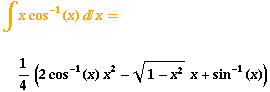Search IntMath
Close

450+ Math Lessons written by Math Professors and Teachers

5 Million+ Students Helped Each Year

1200+ Articles Written by Math Educators and Enthusiasts

Simplifying and Teaching Math for Over 23 Years

# The Integrator

By Murray Bourne, 24 Oct 2007

Have you ever tried The Integrator from Wolfram?

You can input an indefinite integral and The Integrator outputs the answer as an image:There are other output options, including a text-based 'input form' for use in other calculations:

(-(x*Sqrt[1 - x^2]) + 2*x^2*ArcCos + ArcSin)/4

The Integrator uses webMathematica technology to bring the computational capabilities of Mathematica to the web.

This is what happens:

When you type in an integral, The Integrator uses webMathematica to send the integral to the Mathematica kernel, which computes the result using the Integrate function. The result is then embedded into the web page as typeset output.

I wrote recently about the need to improve math notation because the current mess is very confusing for students.

Mathematica's notation would be a good starting point for this improvement (as suggested by David in a comment in that previous post).

Example: Type Sqrt[Sin] for the square root of the sine of x.

• Arguments of functions are put inside square brackets.
• Multiplication is indicated by a space.

I would like to include the Integrator on my Interactive Mathematics site, but the costs are rather prohibitive.

So go ahead and throw an integral at The Integrator. But remember, not every expression can be integrated.

### 3 Comments on “The Integrator”

1. Jackie says:

Very cool - thanks!

2. Darmok says:

That is remarkable!

Good thing that wasn't available back when I was taking calculus...

3. Murray says:

Why, Darmok? Do you mean that you would have used it to get all your answers - and it would have felt like 'cheating'?

### Comment Preview

HTML: You can use simple tags like <b>, <a href="...">, etc.

To enter math, you can can either:

1. Use simple calculator-like input in the following format (surround your math in backticks, or qq on tablet or phone):
a^2 = sqrt(b^2 + c^2)
(See more on ASCIIMath syntax); or
2. Use simple LaTeX in the following format. Surround your math with $$ and $$.
$$\int g dx = \sqrt{\frac{a}{b}}$$
(This is standard simple LaTeX.)

NOTE: You can mix both types of math entry in your comment.